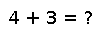# :@evalpoly

@evalpoly(z, c...)

Evaluate the polynomial $\sum_k c[k] z^{k-1}$ for the coefficients c, c, ...; that is, the coefficients are given in ascending order by power of z. This macro expands to efficient inline code that uses either Horner's method or, for complex z, a more efficient Goertzel-like algorithm.

## Examples

Checking you are not a robot: